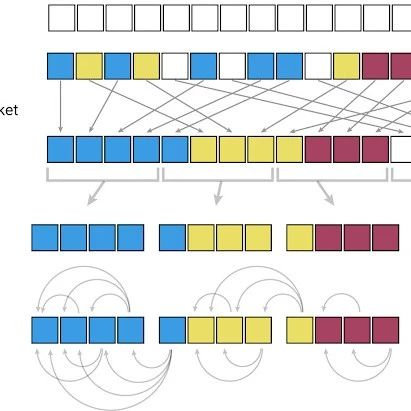注册    登录

 普及数据思维，传播数据文化数据派THU  ·  原创 | ...·  5 天前

# 新年Flag：搞定Python中的“功夫熊猫”，做最高效的数据科学家

Python是一门开源编程语言，使用起来非常方便，但同时也存在一些开源语言固有的问题：实现一个功能有很多库可以用。对于刚入门的Python小白来说，很难知道为实现某个特定功能调用哪个库最好。这时候，就需要有经验的人来提点一下。本文就打算告诉你：有这样一个库，它是所有数据科学从业人员必须掌握的，这个库就叫“pandas”。

Pandas最有趣的地方就是它包含了许多其他Python库的功能，也就是说pandas是各种库的集大成者。这意味着，很多时候你只需要pandas就可以完成大部分工作。

Pandas就像是Python中的Excel：它的基本数据结构是表格（在pandas中叫“DataFrame”），可以对数据进行各种操作和变换。当然，它还能做很多其他的事。

``import pandas as pd``

Pandas中的入门级函数

``data=pd.read_csv('my_file.csv')``
``data=pd.read_csv(my_file.csv',sep=';",encoding="latin-1',nrows=1000,skiprous=[2,5])``

sep参数的意思就是分隔符。如果你要处理的是法语数据，Excel中使用的csv分隔符是“;”，那么你需要通过这个参数显式地声明分隔符。encoding参数需要设置为“latin-1”以便能识别出法语的字符；n_rows=1000表示读取前1000行数据；skiprows=[2,5]的意思是在读取文件时去掉第2行和第5行的数据。

``data.to_csv("my_new_file.csv",index=None)``

``data.shape``

``data.describe()``

``data.head(3)``

``data.loc``

``data.loc[8,'column_1']``

``data.loc[range(4,6)]``

Pandas中的基本函数

``data[data['coluan_1'].isin(["french','engllsh'])]``

``data['column_numerical'].plot()``

.plot()函数的输出示例

``data['column_numerical'].hist()``

.hist()函数的输出示例

``%matplotlib inline``

``data.loc[8,'column_1']='english'``

``data.loc[data['column_1']=='french','column_1']='French'``

``data['column_1'].map(1en)``

len()函数会应用到’column_1’列下的每一个元素。

.map()操作会将一个函数应用到指定列的每一个元素。

``````

data['column_1']. map(1en). map(1ambda x:x/100).plot()``````

Pandas库中一个非常好用的功能就是链式方法。它能够帮助你通过一行代码完成多个操作（比如这里的.map()函数和.plot()函数），既简单又高效。

chaining method（链式方法）:

https://tomaugspurger.github.io/method-chaining

apply函数会将一个函数应用到所有列。

applymap ()函数会将一个函数应用到表格的所有单元。

tqdm — 独一无二的模块

``from tqdm import tqdm_notebook``
``tqdm_notebook().pandas()``

``data['column_1'].progress_map(lambda x:x.count('e"))``

``data.corr()``
``data. corr(). applymap(lambda x: int(x*100)/100)``

``pd.plotting.scatter_matrix(data,figsize=(12,8))``

Pandas中的高级操作

SQL的连接功能

```

`data.merge(other_data,on=['column_1','column_2','column_3'])````

``data.groupby('column_1)['column_2'].apply(sum).reset_index()``

``dictionary={}``
``for i, row in data. iterrows():``
``dictionary[row['column_1']]=row['column_2]``

.iterrows()函数同时获取2个变量并实现循环：分别是行的索引和行的对象（也就是上面代码中的i和row）。

• 方便易用，将所有复杂和抽象的运算过程都隐藏起来；

• 大部分功能的实现方式都非常直观；

• 快速，尽管并不是最快的数据分析库（在C语言中进行了优化）。

Pandas是一个非常重要的工具，它能够帮助数据科学家快速地阅读和理解数据，更高效地完成自己的工作。

https://towardsdatascience.com/be-a-more-efficient-data-scientist-today-master-pandas-with-this-guide-ea362d2738610 小时前4 天前2 天前5 天前6 天前1 周前4 天前4 天前5 天前6 天前